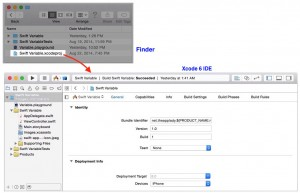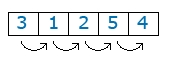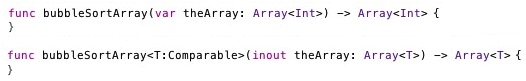A generic function is a function that can take parameters of any type and perform some operation with them. Swift Array and Dictionary use generic. Generic solve the problem of creating separate functions that basically perform the same operation; but with a different type.

For example; say you wanted to sort  an array of string values or an array of Int values; you’ed have to create two separate functions. Instead of doing that, you create a generic function that will sort any type of data you through at it. For example, Int, String, Double, or Character data.

 Before you begin, I assume you have done the following: Read workshop 1-25 Launched the project, Swift Variable.xcodeproj in Xcode 6 IDE-see image below#### Bubble Sort Algorithm

Now that you know what a generic function is and what problem it solve. You are ready to see it in action; but first you need some background information on bubble sort algorithm.

A bubble sort will compare two adjacent elements of an array and will swap them if they are out of order. The compare starts with the first and second element. After that it will compare the second with the third element and so on. The process continues until the end of the list is reached.Figure 1: How bubble sort work to sort elements in an array

When the end of the array is reached, the bubble sort algorithm return to element one and starts the process all over again. So, when will the bubble sort algorithm stop? The bubble sort algorithm knows when it’s finish, when there are no more “swaps”. Take a look at this animated image to see how the bubble sort work.

#### Bubble Sort Function

As you can see in the Swift source code below, the bubble sort algorithm function is easy to program. The function takes a single argument, which is an unsorted Int array, and returns a sorted version of the Int array.

```func bubbleSortArray(var theArray: Array<Int>) -> Array<Int> {
for var row: Int = theArray.count-1; row > 1; --row {
for var col: Int = 0; col < row; ++col {
if theArray[col] > theArray[col + 1] {
// These statements sort theArray
let index1: Int = col, index2: Int = col+1
let temp = theArray[index1]
theArray[index1] = theArray[index2]
theArray[index2] = temp
}
}
}
return theArray
}
// Use the function
bubbleSortArray([49, 07, 33, 23, 77])```

The final line in above code  call the function. Here’s the output it will generate in the playground Result sidebar panel:
[7, 23, 33, 49,7]

#### Generic Bubble Sort Function

Now that you know how to create a bubble sort function that sort an array of Int values; you are ready to learn how to use a generic function that sort an array of any type; be it an array of Int values or an array of String values.

To transform above Swift function to a generic function, you have to modify it its syntax from the first line shown in the image to the second line.Code placed between the { and the } of the generic version of the bubble sort function stays the same as the original. Now, here is a break-down of the generic bubble sort function’s header syntax.

 T means any type; so T must conform to the Comparable protocol. inout theArray: Array This is the argument of the generic function. It takes an array of any type-be it a String type, a Double, etc. Array This is the return type of the generic function. It will return a sorted array of any type. Fore example; if an array of type Int is passed to the function, it will return a sorted version of the Int array back to its caller.

Here is the Swift code to implement the generic bubble sort function:

```func bubbleSortArray(inout theArray: Array) -> Array {
for var row: Int = theArray.count-1; row > 1; --row {
for var col: Int = 0; col < row; ++col {
if theArray[col] > theArray[col + 1] {
// These statements sort theArray
let index1: Int = col, index2: Int = col+1
let temp = theArray[index1]
theArray[index1] = theArray[index2]
theArray[index2] = temp
}
}
}

// Return the sorted array to the caller of this function
return theArray
}```

Now, here are two ways to use the function; and output you will see in a playground file.

Output
[“Zelda”, “44”, “Georgia”, “James”, “!”]
[77, 49, 7, 3, 23, 58]

[“44”, “!”, “Georgia”, “James”, “Zelda”]
[3, 7, 23, 49, 58, 77]

Categories:

Tags:

#### No responses yet

###### UIDocument Demystified: A Step-by-step Guide on Local and iCloud Document Storage